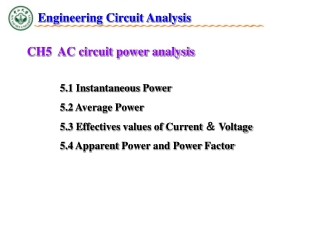Download PresentationCH5 AC circuit power analysis

# CH5 AC circuit power analysis

Download Presentation## CH5 AC circuit power analysis

- - - - - - - - - - - - - - - - - - - - - - - - - - - E N D - - - - - - - - - - - - - - - - - - - - - - - - - - -
##### Presentation Transcript

1. Engineering Circuit Analysis CH5 AC circuit power analysis 5.1 Instantaneous Power 5.2 Average Power 5.3 Effectives values of Current ＆ Voltage 5.4 Apparent Power and Power Factor

2. Ch5 AC circuit power analysis 5.1 Instantaneous Power Given instantaneous power current ＆ voltage, the instantaneous power delivered to any devices is : If it is a resistor If it is a inductor If it is a capacitor

3. Ch5 AC circuit power analysis 5.1 Instantaneous Power The total amount of power supplied by the source = total amount of power delivered to the circuit elements Known Power supplied by the source Power delivered to R: Power delivered to L: PL(t) = VL(t) ∙ iL(t) R + - V0 + -

4. Ch5 AC circuit power analysis 5.1 Instantaneous Power Since

5. Ch5 AC circuit power analysis 5.1 Instantaneous Power How if both ＆ sinusoidal signals? It is known , leading to possible to be the averaged power? twice of Time invariant

6. ) + ~ - Ch5 AC circuit power analysis 5.1 Instantaneous Power • Example 8.1 Given a voltage source of 40 + 60μ(t) V, a 5 μF capacitor and a 200 Ω resistor being connected in series. Find at t = 1.2ms, the powers being absorbed by the resistor and capacitor respectively. If PS = PC + PR? When t<0 ,Vs=40V When t=0 , Vs=100V , and Hence In this 1st order RC circuit Hence ,

7. Ch5 AC circuit power analysis 5.1 Instantaneous Power The average power (over time) for the instantaneous power The average power over time intervals to --If is periodic as , where is the period Validate the previous suggestion !

8. Ch5 AC circuit power analysis 5.1 Instantaneous Power • Example : the averaged power for It is a periodic signal with a period of Phase different between ＆ ,or Validate the previous suggestion !

9. Ch5 AC circuit power analysis 5.2 Average Power Two observations: -- power absorbed by an ideal resistor, -- power absorbed by a purely reactiveelement, , Example 8.2 (p.213) Given and Determine P and p(t).

10. ) + + ~ ~ - - Ch5 AC circuit power analysis 5.2 Average Power Apply KVL, we can determine the currents are: The current that passes through the resistor is: Hence , Both L & C are reactive elements , and For the source on the left mesh For the source on the right mesh The power of the left supplies 50W power and it is absorbed by the resistor and the source on the right Example 8.4 Determine the average power absorbed by each of the passive elements and suppliedby each of the sources.

11. Ch5 AC circuit power analysis 5.2 Average Power P

12. Ch5 AC circuit power analysis 5.2 Average Power Remark : superposition is applicable for evaluating the averaged power of a non periodic function which can be decomposed into two periodic functions with different periods,

13. Ch5 AC circuit power analysis 5.2 Average Power • Example 8.6 (p218) Determine the average power delivered to the 4 Ω resistor by the current i1 = 2 cos10t – 3 cos20t A. The two different frequency components of the current can be considered separately. i1 = 2 cos10t – 3 cos20t A

14. Ch5 AC circuit power analysis 5.3Effectives values of Current ＆ -- represent the actual effect of a periodic function (current voltage ) in term of delivering / absorbing power -- transforms an AC circuit into an equivalent DC circuit -- square root of a mean square (RMS)

15. Ch5 AC circuit power analysis 5.3Effectives values of Current ＆ RMS of the Sinusoidal wave. Given , For a sinusoidal function , its RMS is of its magnitude

16. Ch5 AC circuit power analysis 5.3Effectives values of Current ＆ Effective value of multiple-frequency circuit Power can be determined using super position of 50Hz of 100Hz In general, given a circuit with frequencies,

17. Ch5 AC circuit power analysis 5.3Effectives values of Current ＆ Example …

18. Ch5 AC circuit power analysis 5.4 Apparent Power and Power Factor Given One can determine the averaged power delivered to the network is or However, the effective power delivered to the network would be is defined as the apparent power – The maximal value of the average power can be. Note that the unit of the Papp is VA.

19. , capacitive load, since lags behind , inductive load, since leads , a leading PF of “—current is leading” a lagging PF of “—current is lagging” Ch5 AC circuit power analysis 5.4 Apparent Power and Power Factor Power factor ( ) In the above case, If passive elements are all resistive, If passive elements are all reactive, , If passive elements are neither purely resistive, nor purely reactive

20. + - + - Ch5 AC circuit power analysis 5.4 Apparent Power and Power Factor Example 8.8(P222) Determine the apparent power supplied by the source3, and the power factor of the combined loads. Note that for the impedance 3+j4Ω,only the resistivecomponentwill absorb power delivered by the source. ad